Following this week's puzzle in New York Times wordplay blog I found out about Dr. Nelson Blachmanan amazing mathematician. Producing mathematical research is difficult, let alone for Dr. Blachman who is 90 years old this year and also blind, however, he is still an active mathematician, an expert user of Mathematica and uses computers without a glitch (video).

Dr Blachman will publish his solution to the Gambling Machine puzzle on the 11th of March 2013, however, I was interested to approach that problem again from a computational point of view and give you a heads up while anticipating his solution. You can also have a look on the comments of the wordplay blog to see other approaches as well. Please read mine and if you find any mistakes or have any objections, I will be more than happy to further discuss it. My code is available here or you can clone it with

git clone This email address is being protected from spambots. You need JavaScript enabled to view it.:gpierris/the-gambling-problem.git

The Gambling Machine puzzle as described in New York Times wordplay blog considers "a gambling machine that chooses two independent random variables x and y that are uniformly and independently distributed between 0 and 100." The value of x becomes known to the customer who then guesses whether y is larger or smaller than x. If you guess correctly then the amount of y dollars is given back to you, if x=y then y/2 is given, and otherwise you are not given anything. Finally, the price of the ticket to take part in the game is 40 dollars.

## Understanding the problem

There are at least two kinds of players that I am interested to analyse, namely, the rational player who carefully guesses based on the known value of x, and the random player who randomly guesses the relationship of x and y. The rational player understands basic principles of probability theory and quickly realizes that if x is less than 50, then it is highly probable that y will be larger than x, i.e., the "x<y" case, and vice versa. For example, if x is 30 then the probability of y to be larger is 70/101 (we divide by 101 because we include zero) compared to 30/101 for smaller and 1/101 to be equal.

With the fixed policy of the rational player, if we assume that the mean value of x will be either 25 for the cases that it is less than 50 and 75 for the cases that is above 50, we expect that the probability of successfully guessing the relationship of x and y will be around 75%.

Having successfully guessed, the mean value of the expected earnings will be (25+...+100) / 76 = 62.5 dollars on the "x<y" case, which is found by calculating the mean value of the possible values of y. On the "x>y" case, the mean earnings of our player drop down to (0+...+75) / 76 = 37.5 dollars. Assuming that both events are equipossible we expect the mean earnings to balance at the 50 dollars. Combining the fact that we can be around 75% successful in our predictions and having mean earnings of 50 dollars, we expect to have a return at each guess of 50*75% = 37.5 dollars. Please remember that in the above calculations I have loosely and quietly ignored the case of "x=y" which occurs around 1 time every 100 attempts and results in earnings of y/2.

Finally, considering that we pay a ticket of 40 dollars for the privilege to play the game, then I would say it is unfair for the customer, nevertheless, let's simulate it and see the results!

## Computational Experiments

### Case 1

To begin with, I should computationally verify the aforementioned claims and calculations. In a first experiment, I run one long episode with 1000 attempts for the same customer. As seen from the figure below, indeed the probability of successfully guessing converges to 75%. In particular, we had 758 wins over 242 losses, i.e., a probability of 75.8% . It is worth mentioning that the case "x=y" is treated as a successful prediction, even though the earnings are half. As expected, the random customer on the other hand has a converged probability of winning of 53.3. If you are unsatisfied with the 53.3%, in another run with 10000 attempts this probability converges to 50.03%.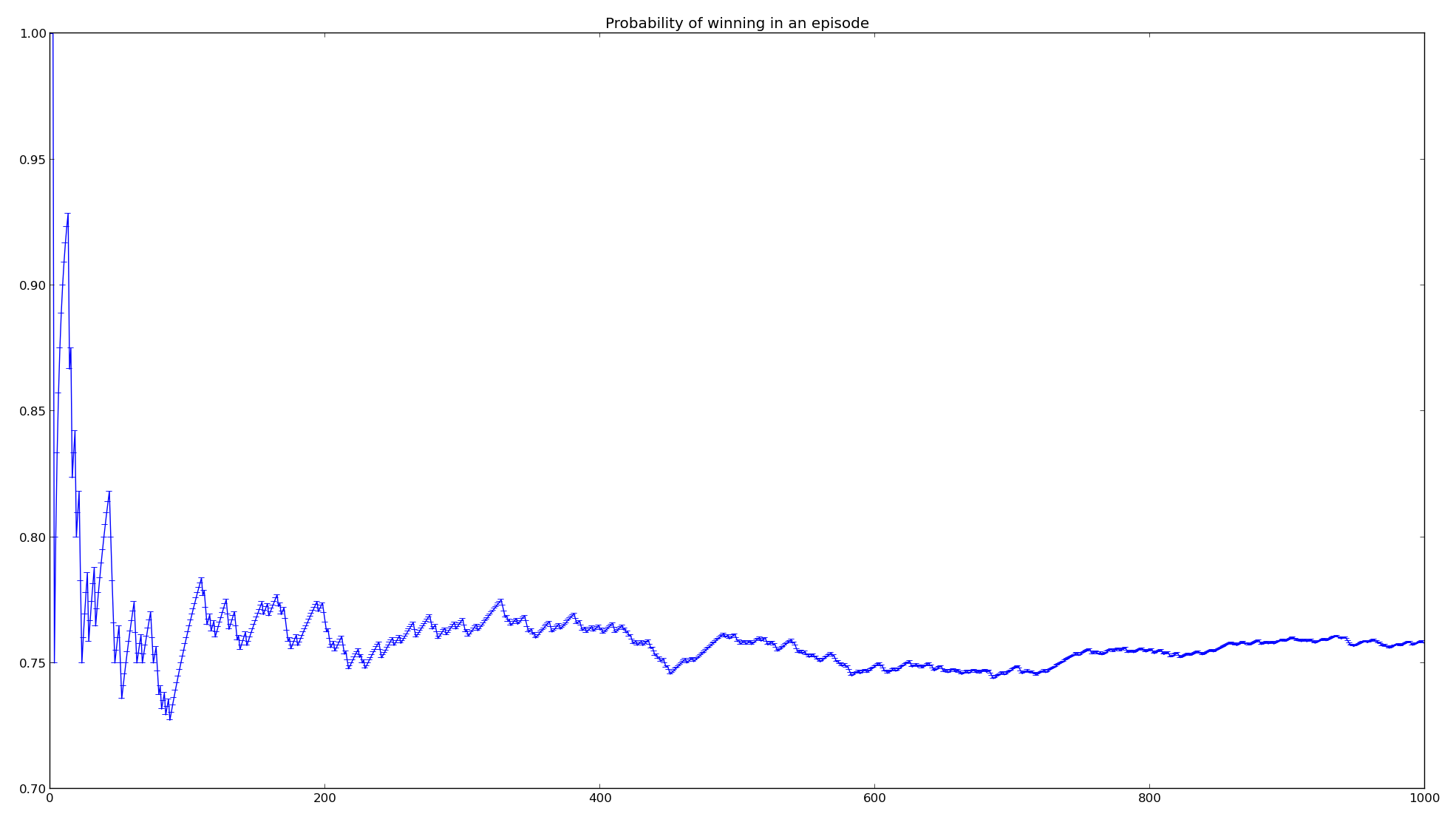We should also verify that the mean value of earnings is close to 50 dollars. However, without ignoring the cases that "x=y" where we only earn y/2, we should expect the earnings to converge at just below 50 dollars. Indeed, the same simulation reported a mean value of earnings of 50.56 dollars and for 10000 attempts it goes down to 49.80.  Considering the "x=y" case, the mean value of earnings should be 50*(100/101) plus the half value of the expected earning, i.e., 50/2=25, one time every 101 tries that x happens to be equal to y, so 50*(100/101)+25*(1/101) = 49.75 dollars.

Below you can see the decreasing cash flow for the rational (in blue) and random (in green) customer.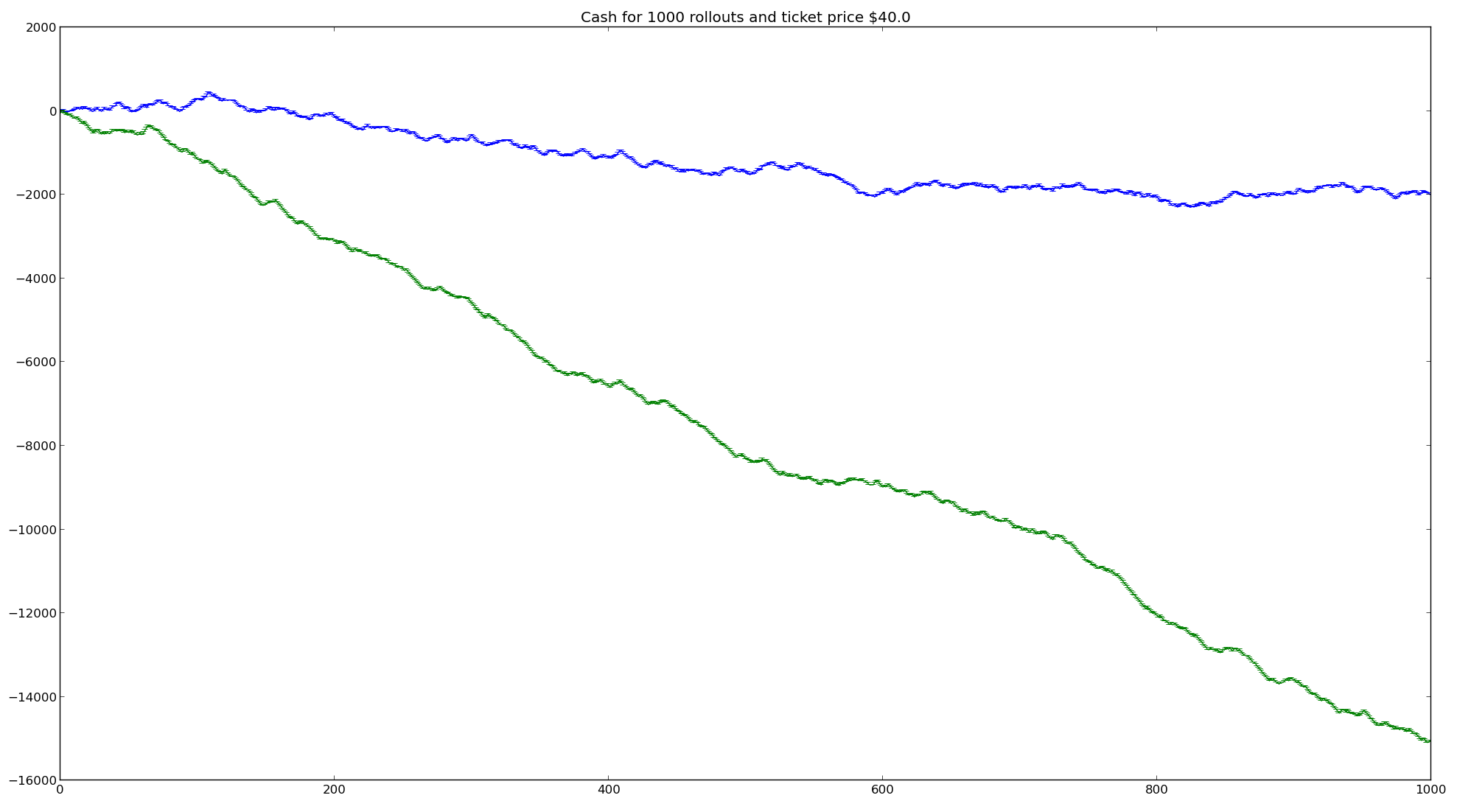### Case 2

Case 1 reflects the results for the customer that had 1000 attempts which requires to spend 40000 dollars in that game. A bit odd for real life. Therefore, I devise a different experiment where multiple episodes, e.g., 10000 different customers, give the game a try for 100 times each. As we can see from the figures below the probability of winning converges again to 75% and additionally we observe that the deviation is also decreasing.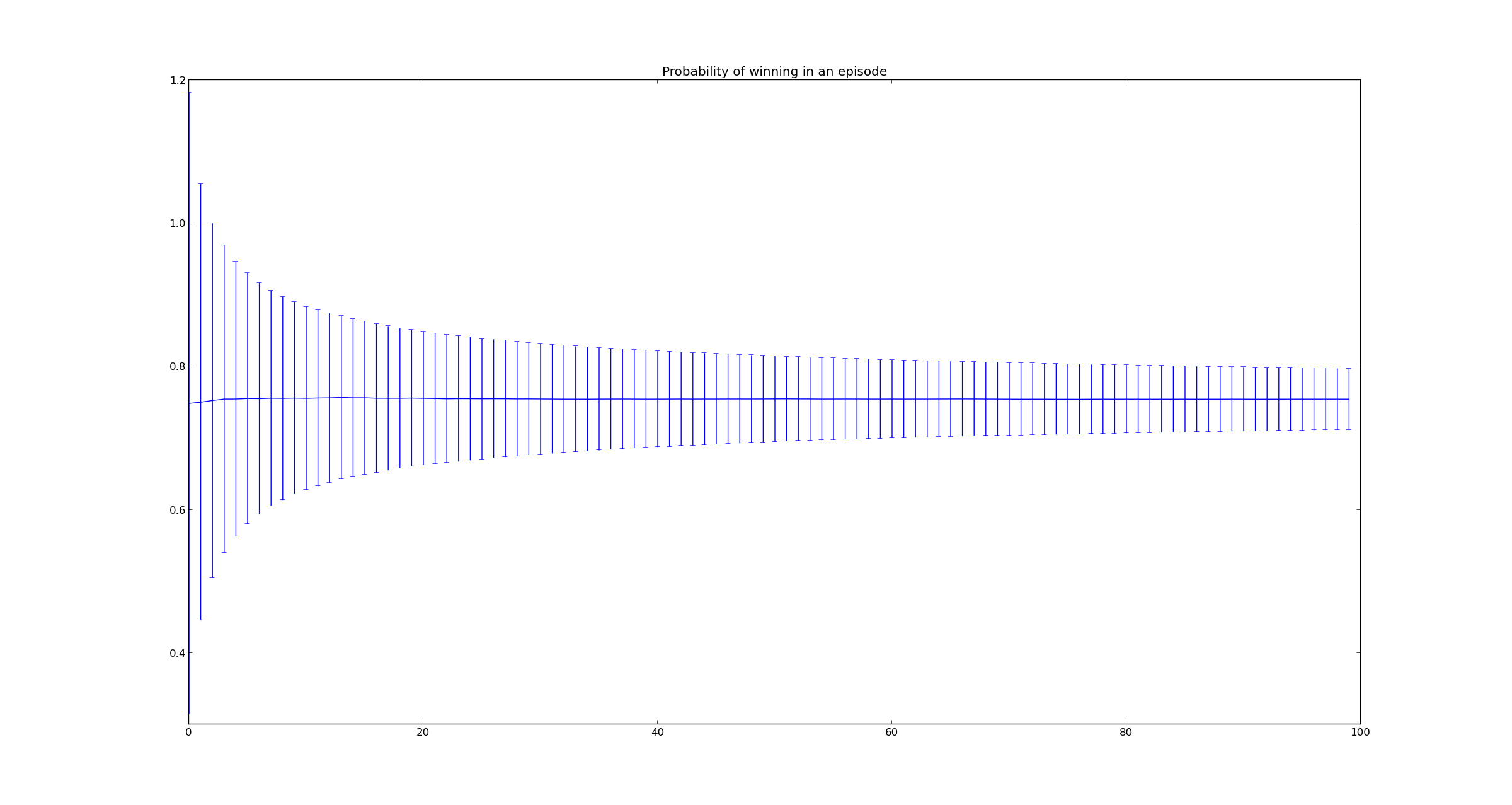Below you can see that the mean value of cash flow of both the rational customer (in blue) and the random customer (in green) are also decreasing with high deviations. These deviations suggest that the lucky one will have some earnings, however, it is unlikely that the game will be profitable for the customer in the long run. For the random player the drop is drastic.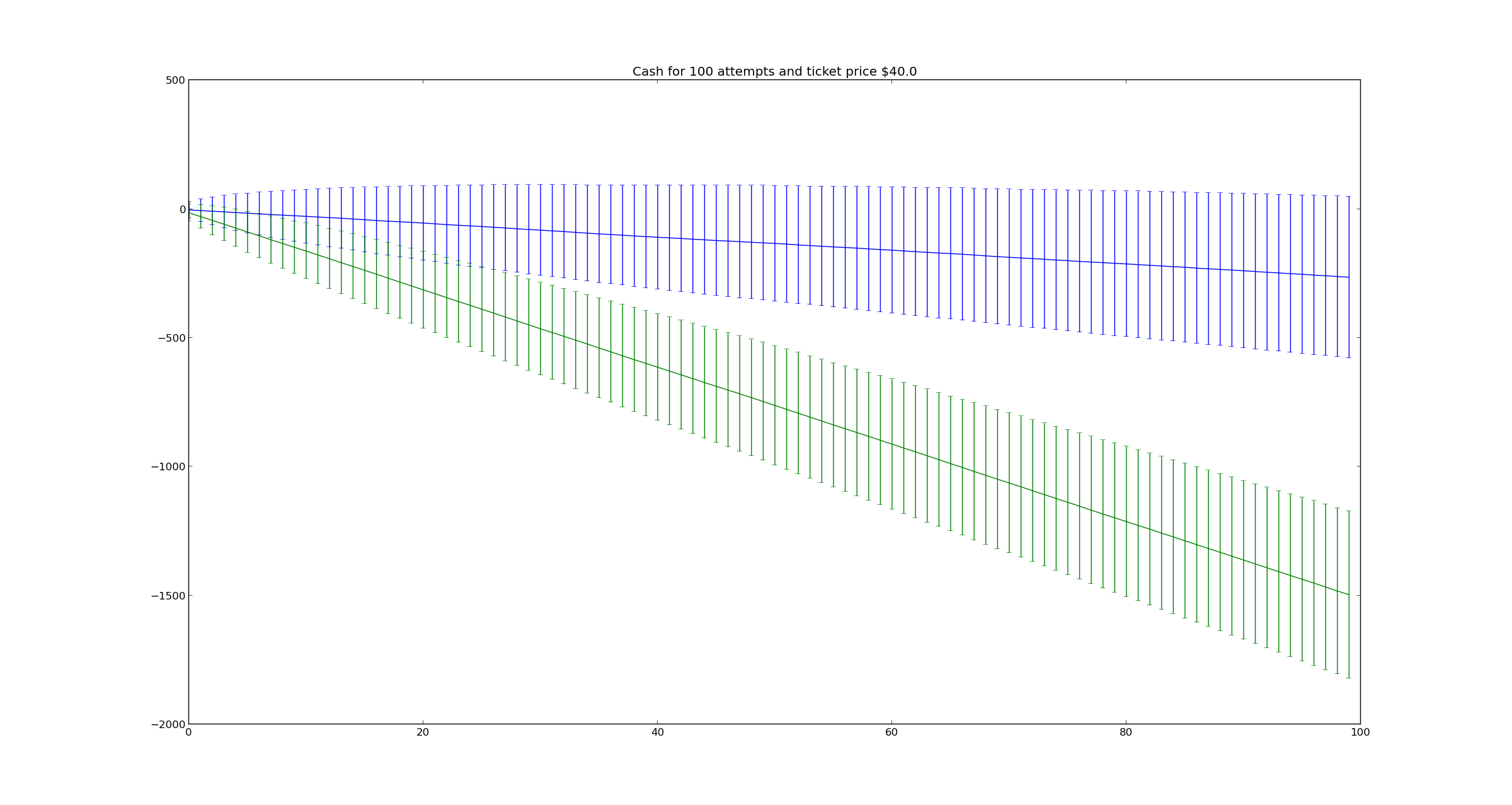### Case 3

According to our theory we should expect that the rational player is winning 37.5 dollars per attempt, however, he gives 40 dollars for the ticket. To verify this, if we set the ticket to 37.5 dollars, then we should see the mean cash flow of the customer to be constant around zero. Let's see if we are correct in the graph below.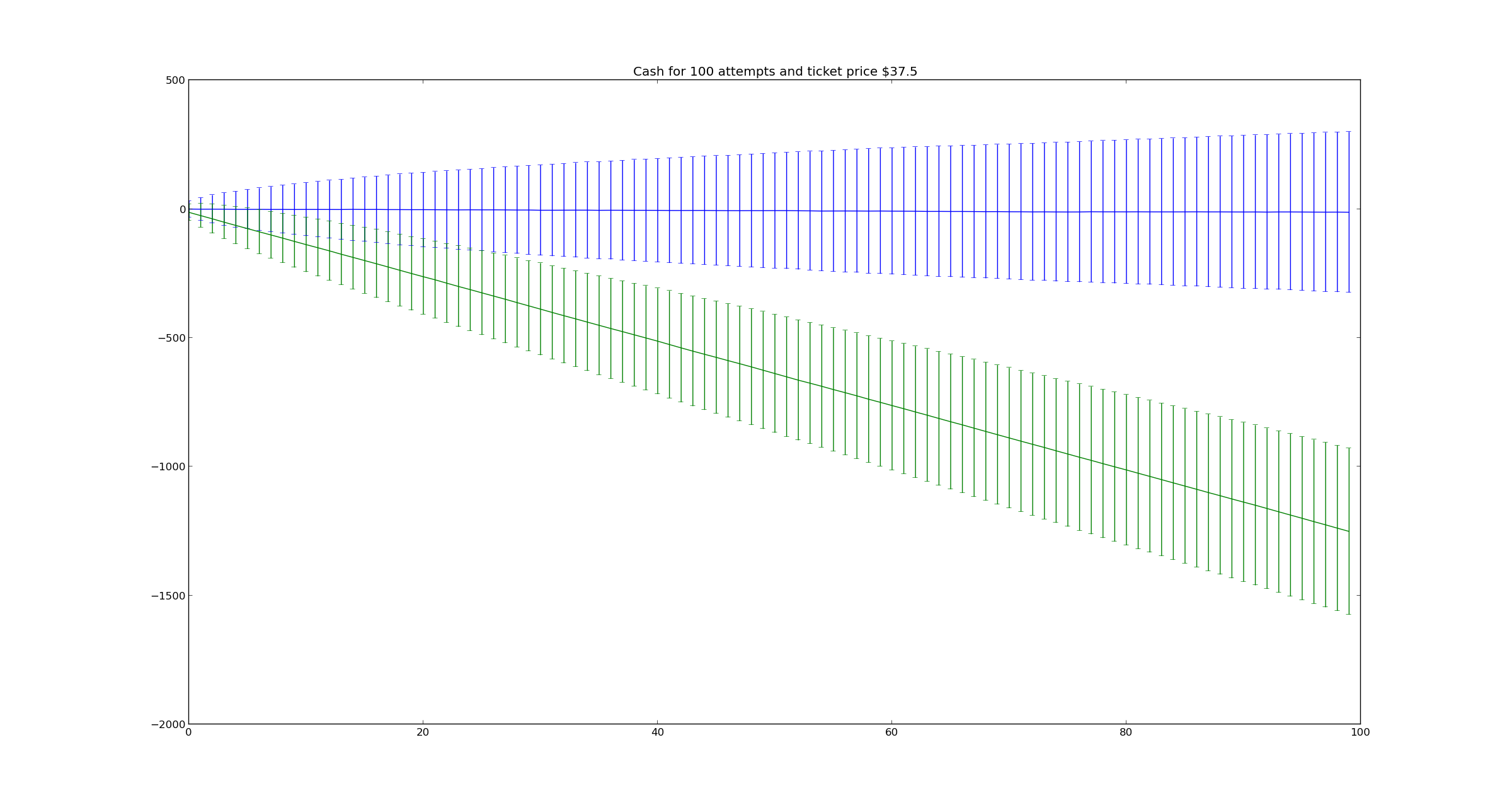### Case 4

For any ticket price below 37.5, then I would bet my money. Have a look below for a ticket of 35 dollars. The random player still wastes time and money.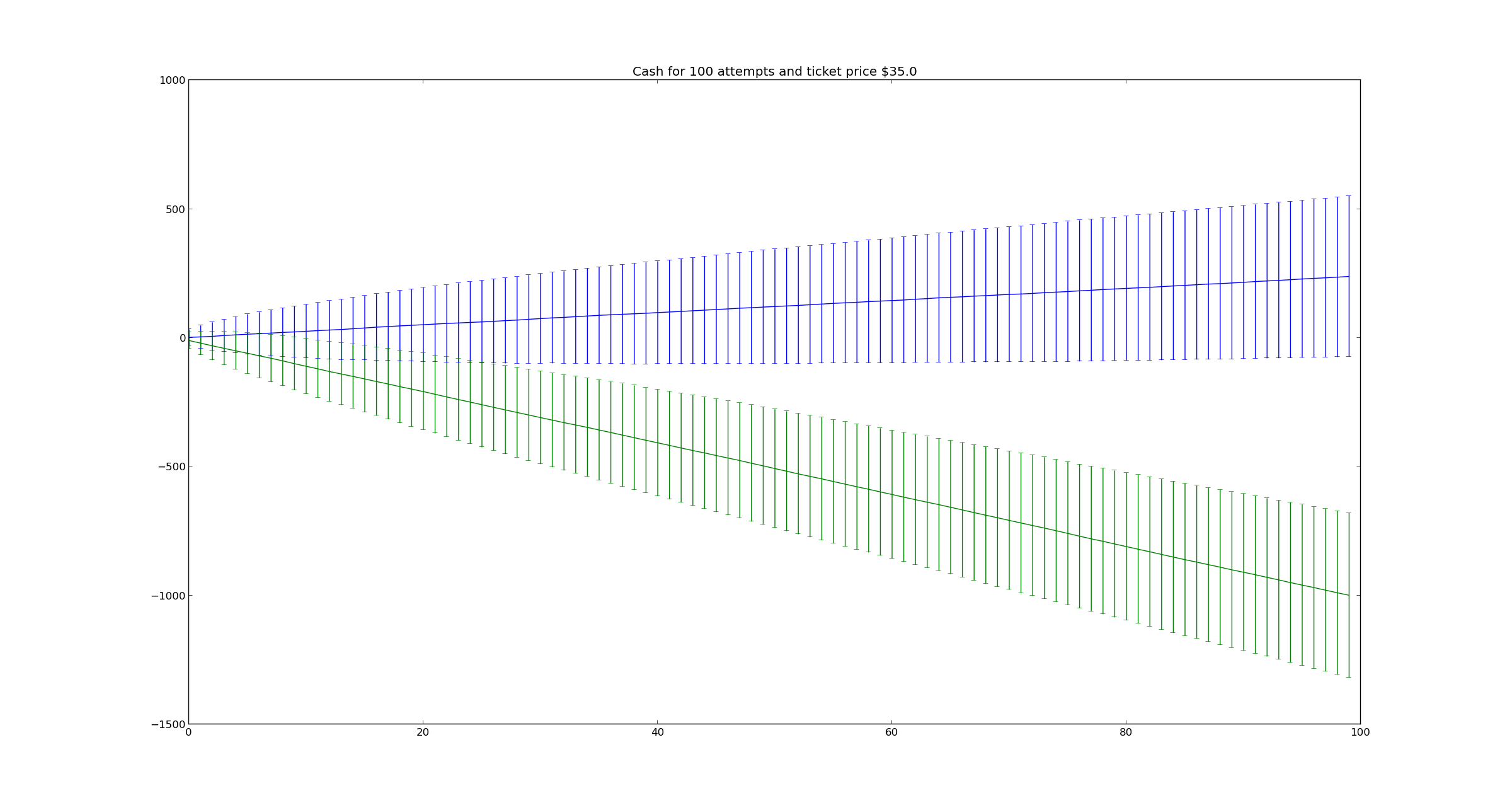### The verdict

Well, the verdict should be clear by now. You shouldn't play this game with a ticket price of 40 dollars. However, if you are really lucky then you should not be bothered to read this article anyway.